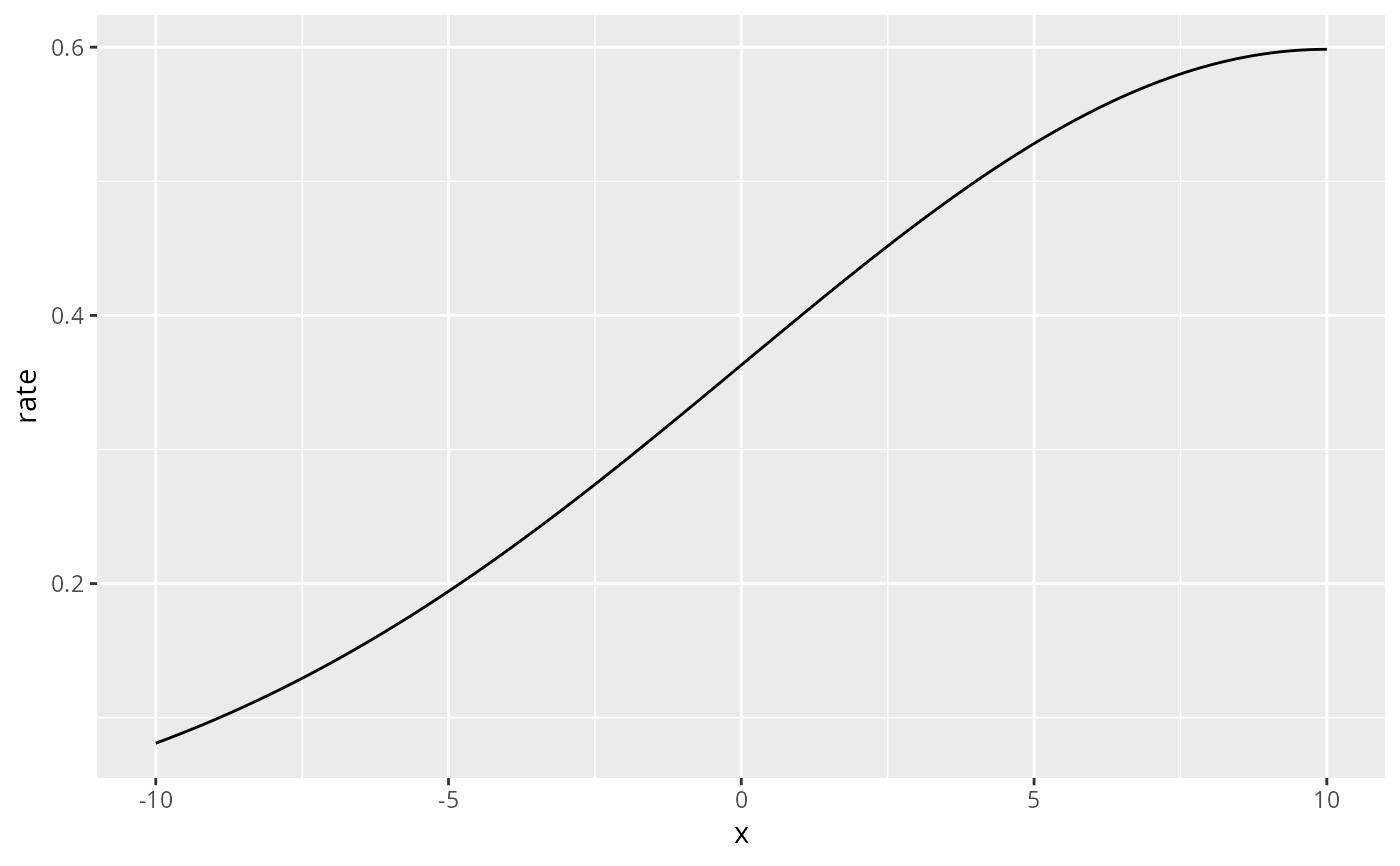This data set serves to teach the concept of modelling species that gather in groups and where the grouping behaviour depends on space.

## Usage

data(toygroups)

## Format

The data are a list that contains these elements:

groups:

A data.frame of group locations x and size size

df.size:

IGNORE THIS

df.intensity:

A data.frame with Poisson process intensity d.lambda at locations x

df.rate:

A data.frame the locations x and associated rate which parameterized the exponential distribution from which the group sizes were drawn.

## Examples

# \donttest{
if (require(ggplot2, quietly = TRUE)) {

data("toygroups", package = "inlabru")

# The data set is a simulation of animal groups residing in a 1D space. Their
# locations in x-space are sampled from a Cox process with intensity

ggplot(toygroups$df.intensity) + geom_line(aes(x = x, y = g.lambda)) # Adding the simulated group locations to this plot we obtain ggplot(toygroups$df.intensity) +
geom_line(aes(x = x, y = g.lambda)) +
geom_point(data = toygroups$groups, aes(x, y = 0), pch = "|") # Each group has a size mark attached to it. # These group sizes are sampled from an exponential distribution # for which the rate parameter depends on the x-coordinate ggplot(toygroups$groups) +
geom_point(aes(x = x, y = size))

ggplot(toygroups\$df.rate) +
geom_line(aes(x, rate))
}# }